# The RAID-6 Liberation Codes

James S. Plank
Department of Electrical Engineering and Computer Science
University of Tennessee

## Abstract

The RAID-6 specification calls for a storage system with multiple storage devices to tolerate the failure of any two devices. Numerous erasure coding techniques have been developed that can implement RAID-6; however, each has limitations. In this paper, we describe a new class of RAID-6 codes called the Liberation Codes. These codes encode, update and decode either optimally or close to optimally. Their modification overhead is lower than all other RAID-6 codes, and their encoding performance is often better as well. We provide an exact specification of the Liberation Codes and assess their performance in relation to other RAID-6 coding techniques. In the process, we describe an algorithm called bit matrix scheduling, which improves the performance of decoding drastically. Finally, we present a freely available library which facilitates the use of Liberation Codes in RAID-6 sytems.

# 1 Introduction

As storage systems have grown in size and complexity, applications of RAID-6 fault-tolerance have become more pervasive. RAID-6 is a specification for storage systems composed of multiple storage devices to tolerate the failure of any two devices. In recent years, RAID-6 has become important when a failure of one disk drive occurs in tandem with the latent failure of a block on a second drive [Corbett04]. On a standard RAID-5 system, this combination of failures leads to permanent data loss. Hence, storage system designers have started turning to RAID-6.

Unlike RAID-1 through RAID-5, which detail exact techniques for storing and encoding data to survive single disk failures, RAID-6 is merely a specification. The exact technique for storage and encoding is up to the implementor. Various techniques for implementing RAID-6 have been developed and are based on erasure codes such as Reed-Solomon coding [Anvin07,Reed60], EVENODD coding [Blaum95] and RDP coding [Corbett04]. However, all of these techniques have limitations -- there is no one de facto standard for RAID-6 coding.

This paper offers an alternative coding technique for implementing RAID-6. We term the technique The RAID-6 Liberation Codes, as they give storage systems builders a way to implement RAID-6 that frees them from problems of other implementation techniques. We give a complete description of how to encode, modify and decode RAID-6 systems using the Liberation Codes. We also detail their performance characteristics and compare them to existing codes.

The significance of the Liberation Codes is that they provide performance that is optimal, or nearly optimal in all phases of coding. They outperform all other RAID-6 codes in terms of modification overhead, and in many cases in encoding performance as well. We provide a freely available library that implements the various pieces of Liberation Coding. As such, we anticipate that they will become very popular with implementors of RAID-6 systems.

# 2 RAID-6 Specification and Current Implementations

RAID-6 is a specification for storage systems with k+2 nodes to tolerate the failure of any two nodes. Logically, a typical RAID-6 system appears as depicted in Figure 1. There are  k+2 storage nodes, each of which holds  B bytes, partitioned into  k data nodes, D0, ... Dk-1, and two coding nodes P and Q. The entire system can store  kB bytes of data, which are stored in the data nodes. The remaining 2B bytes of the system reside in nodes P and Q and are calculated from the data bytes. The calculations are made so that if any two of the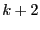nodes fail, the data may be recovered from the surviving nodes.Actual implementations optimize this logical configuration by settingto be smaller than each disk's capacity, and then rotating the identity of the data and coding devices everybytes. This helps remove hot spots in the system in a manner similar to RAID-5 systems. A pictoral example of this is in Figure 2. For simplicity, in the remainder of this paper we assume that each storage node contains exactlybytes as in Figure 1 since the extrapolation to systems as in Figure 2 is straightforward.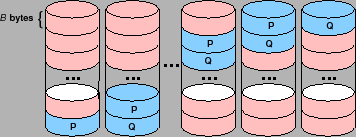The P device in RAID-6 is calculated to be the parity of the data devices. In this way, RAID-6 systems extrapolate naturally from RAID-5 systems by simply adding a Q drive. It also means that the sole challenge in designing a RAID-6 coding methodology lies in the definition of the Q drive. This definition must result in a maximum distance separable (MDS) code, which means that the Q drive cannot hold more than B bytes, and the original data must be restored following the failure of any two of the k+2 devices.

There are several criteria that a storage system designer must evaluate when selecting an erasure coding technique for a RAID-6 system:

• Encoding performance is the speed of calculating P and Q from D0 ... Dk-1.
• Modification performance is the speed of recomputing relevant parts of P and Q when one of the's is modified.
• Decoding performance is the speed of recalculating lost data or coding information following one or two failures.
• Ease of Implementation is the complexity of the technique.
• Cost of Implementation pertains to licensing issues, as many erasure coding techniques are patented.

Below, we detail current techniques for implementing RAID-6.

Reed-Solomon Coding [Reed60] is a very powerful general-purpose coding technique. It involves breaking up the data on each device into w-bit words, and then having the i-th word on the Q device be calculated from the i-th word on each data device using a special kind of arithmetic called Galois Field arithmetic (GF(2w)). Galois Field addition is equivalent to the XOR operation; multiplication is much more difficult and requires one of a variety of techniques for implementation. As such, Reed-Solomon Coding is expensive compared to the other techniques. Reed-Solomon Coding is described in every text on coding theory [MacWilliams77,Moon05,Peterson72,vanLint82] and has tutorial instructions written explicitly for storage systems designers [Plank97,Plank05].

Reed-Solomon Coding for RAID-6: Recently, Anvin has described a clever optimization to Reed-Solomon encoding for RAID-6 [Anvin07], based on the observation that multiplication by two may be implemented very quickly when w is a power of two. This optimization speeds up the performance of Reed-Solomon encoding. It does not apply to modification or decoding.

Parity Array coding applies a different methodology which is based solely on XOR operations. It works logically on groups of w bits from each data and coding device. The data bits of device Di are labeled  di,0, ..., di,w-1, and the coding bits are  p0, ..., pw-1 and  q0, ..., qw-1 for the P and Q devices respectively. The p bits are calculated to be the parity of their respective data bits:The q bits are defined to be the parity of some other collection of the data bits, and this definition is what differentiates one parity array code from another. A parity array system for k=5 and w=4 is depicted in Figure 3.Obviously, to be efficient from an implementation standpoint, parity array codes do not work on single bits, but instead on w groups of bytes per RAID-6 block. In this way, we are not performing XORs on bits, but on machine words, which is very efficient. Thus the block size B defined above is restricted to be a multiple of w and the machine's word size.

Cauchy Reed-Solomon Coding is a technique that converts a standard Reed-Solomon code in GF(2w) to a parity array code which works on groups of w bits [Blomer95]. This has been shown to reduce the overhead of encoding and decoding [Plank06], but not to the degree of the codes that we describe next.

EVENODD coding departs from the realm of Reed-Solomon coding by defining the qi bits from diagonal partitions of the data bits [Blaum95]. We do not provide an exact specification, but to give a flavor, we show the EVENODD code for k=5 and w=4 in Figure 4 (since the P device is parity, we do not picture it). The value S is an intermediate value used to calculate each qi.The parameter w must be selected such that w+1 ≥ k and w+1 is a prime number. Although this gives storage designers a variety of w to choose from for a given value of k, smaller w are more efficient than larger w.

EVENODD coding performs significantly better than all variants of Reed-Solomon coding. Its encoding performance is roughlyXOR operations per coding word. Optimal encoding is equal to k-1 XOR operations per coding word [Blaum99,Xu99]. Its modification performance is roughly three coding words per modified data word. Optimal is two. Finally, its decoding performance is roughlyXOR operations per failed word. As with encoding, optimal decoding performance isXOR operations per failed word. Thus, EVENODD coding achieves performance very close to optimal for both encoding and decoding. EVENODD coding was patented in 1996 [Blaum96].

RDP Coding is a parity array coding technique that is very similar to EVENODD coding, but improves upon it in several ways [Corbett04]. As with EVENODD coding, the number of bits per device, w, must be such that w+1 is prime; however w+1 must be strictly greater than k rather than ≥ k. RDP calculates the bits of the Q device from both the data and parity bits, and in so doing achieves better performance. We show the RDP code for k=4 and w=4 in Figure 5.When w = k or w = k+1, RDP achieves optimal performance in both encoding and decoding. When w ≥ k+2, RDP still outperforms EVENODD coding and decoding, but it is not optimal. Like EVENODD coding, RDP coding modifies roughly three coding bits per modified data bit. RDP coding was patented in 2007 [Corbett07].

There are other very powerful erasure coding techniques that have been defined for storage systems. We do not address them in detail because they do not apply to RAID-6 systems as defined above. However, we mention them briefly. The X-Code [Xu99] is an extremely elegant erasure code for two-disk systems that encodes, decodes and updates optimally. However, it is a vertical code that requires each device to hold two coding words for everydata words. It does not fit the RAID-6 specification of having coding devices P and Q, where P is a simple parity device.

The STAR code [Huang05] and Feng's codes [Feng05A,Feng05B] define encoding methodologies for more than two failures. Both boil down to EVENODD coding when applied to RAID-6 scenarios. There are other codes [Hafner05,Hafner06,Huang07A,Wylie07] that tolerate multiple failures, but are not MDS, and hence cannot be used for RAID-6.

## 2.1 Why Do We Need Another Code?

Simply put, Reed-Solomon Coding is slow, and the parity array coding techniques exhibit suboptimal modification performance and are patented. While patents should not have relevance to academic research papers, they do have a profound impact on those who implement storage systems and are the main reason why RAID-6 systems are still being implemented with Reed-Solomon coding. As such, alternative coding techniques that exhibit near-optimal performance are quite important.

Regardless of the patent issue, the Liberation codes have many properties that make them an attractive alternative to other RAID-6 techniques:

• They are parity array codes whose encoding performance is close to optimal. For all values of k, they outperform EVENODD encoding, and for some values of k, they even outperform RDP encoding. Thus, for many values of k, they represent the best known RAID-6 codes.
• To build flexible RAID-6 systems, it is often advantageous to allow k to grow and shrink dynamically within limits. For the parity array codes (including Liberation codes), this means employing a fixed value of w in all cases that can accomodate the largest possible value of k. EVENODD and RDP coding systems will work in this way, but their performance suffers when k shrinks, because they cannot compensate by decreasing w as well. In contrast, Liberation codes improve as w grows, and thus exhibit better performance in systems where k varies beneath a threshhold value.
• Their modification performance is very close to the optimal value of two updated coding bits per modified data bit. This is an improvement on the other coding techniques, and it can be shown that it achieves the lower bound for all RAID-6 codes.
• The decoding performance is within 15% of optimal.
• Their implementation is freely available.

We describe the codes and analyze their performance below.

# 3 Liberation Code Description

Liberation coding and decoding are based on a bit matrix-vector product very similar to the those used in Reed-Solomon coding [MacWilliams77,Peterson72] and Cauchy Reed-Solomon coding [Blomer95]. This product precisely defines how encoding and modification are performed. Decoding is more complex and to proceed efficiently, we must augment the bit matrix-vector product with the notion of bit matrix scheduling.'' We first describe the general methodology of bit matrix coding and then define the Liberation Codes and discuss their encoding/modification performance. We then describe decoding, and how its performance may be improved by bit matrix scheduling. We compare the Liberation Codes to the other RAID-6 codes in Section 4.

## 3.1 Bit Matrix Coding Overview

Bit matrix coding is a parity array coding technique first employed in Cauchy Reed-Solomon coding [Blomer95]. In general, there are k data devices and m coding devices, each of which holds exactly w bits. The system uses a  w(k+m) by wk matrix over GF(2) to perform encoding. This means that every element of the matrix is either zero or one, and arithmetic is equivalent to arithmetic modulo two. The matrix is called a binary distribution matrix, or BDM. The state of a bit matrix coding system is described by the matrix-vector product depicted in Figure 6.The BDM has a specific format. Its first wk rows compose a  wk by wk identity matrix, pictured in Figure 6 as a  k by k matrix whose elements are each  w by w bit matrices. The next mw rows are composed of mk matrices, each of which is a  w by w bit matrix Xi,j.

We multiply the BDM by a vector composed of the wk bits of data. We depict that in Figure 6 as k bit vectors with w elements each. The product vector contains the (k+m)w bits of the entire system. The first wk elements are equal to the data vector, and the last wm elements contain the coding bits, held in the m coding devices.

Note that each device corresponds to a row of  w by w matrices in the BDM, and that each bit of each device corresponds to one of the w(k+m) rows of the BDM. The act of encoding is to calculate each bit of each Ci as the dot product of that bit's row in the BDM and the data. Since each element of the system is a bit, this dot product may be calculated as the XOR of each data bit that has a one in the coding bit's row. Therefore, the performance of encoding is directly related to the number of ones in the BDM.

To decode, suppose some of the devices fail. As long as there are k surviving devices, we decode by creating a new  wk by wk matrix BDM' from the wk rows corresponding to k of the surviving devices. The product of that matrix and the original data is equal to these k surviving devices. To decode, we therefore invert BDM' and multiply it by the survivors- that allows us to calculate any lost data. Once we have the data, we may use the original BDM to calculate any lost coding devices.

For a coding system to be MDS, it must tolerate the loss of any m devices. Therefore, every possible BDM' matrix must be invertible. This is done in Cauchy Reed-Solomon coding by creating each Xi,j from a Cauchy matrix in GF(2w)  [Blomer95]. However, these do not perform optimally. It is an open question how to create optimally performing bit matrices in general.

Since the first wk rows of the BDM compose an identity matrix, we may precisely specify a BDM with a Coding Distribution Matrix (CDM) composed of the last wm rows of the BDM. It is these rows that define how the coding devices are calculated. (In coding theory, the CDM composes the leftmost wk columns of the parity check matrix).

## 3.2 RAID-6 Bit Matrix Encoding

When this methodology is applied to RAID-6, the BDM is much more restricted. First, m=2, and the two coding devices are named P=C0 and Q=C1. Since the P device must be the parity of the data devices, each matrix X0,i is equal to a  w by w identity matrix. Thus, the only degree of freedom is in the definition of the X1,i matrices that encode the Q device. For simplicity of notation, we remove the first subscript and call these matrices  X0, ..., Xk-1. A RAID-6 system is depicted in Figure 7 for k=4 and w=4.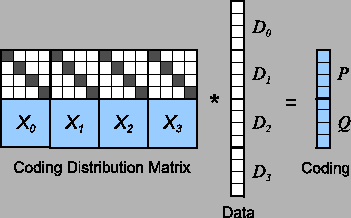To calculate the contents of a coding bit, we simply look at the bit's row of the CDM and calculate the XOR of each data bit that has a one in its corresponding column. For example, in Figure 7, it is easy to see that.

When a data bit is modified, we observe that each data bit di,j corresponds to column wi+j in the CDM. Each coding bit whose row contains a one in that column must be updated with the XOR of the data bit's old and new values.

Therefore, to employ bit matrices for RAID-6, we are faced with a challenge to define the Xi matrices so that they have a minimal number of ones, yet remain MDS. A small number of ones is important for fast encoding and updating. We shall see the impact on decoding later in the paper.It is an interesting aside that any RAID-6 code based on XOR operations may be defined with a bit matrix. To demonstrate, we include the Xi for EVENODD, RDP and Cauchy Reed-Solomon coding when k=6 and w=6 in Figure 8. It is a simple matter to verify that each of these defines an MDS code.

There are 61 ones in the EVENODD matrices, 60 in the RDP matrices and 46 in the Cauchy Reed-Solomon matrices. Thus, were one to encode with the bit matrices, the Cauchy Reed-Solomon coding matrices would be the fastest, which would seem to contradict the fact that RDP encodes optimally. We explore this more fully in Section 3.4 below, where we demonstrate how to improve the performance of bit matrix encoding so that it does not rely solely on the number of ones in the matrix.

The performance of updating, however, is directly related to the number of ones in each column, and there is no way to optimize that further. The fact that EVENODD and RDP coding update must update roughly three coding bits per data bit is reflected in their CDM's, which have an average ofandones per column respectively (we add 36 ones for the identity matrices that encode the P device). The Cauchy Reed-Solomon CDM requires only 2.31 modifications per data bit.

## 3.3 Liberation Code Specification

We now define the Liberation codes. As with EVENODD and RDP coding, the value of w is restricted and depends on k. In particular, w must be a prime number ≥ k and > 2. To specify the Xi matrices, we use two pieces of notation:

• We define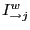to be the  w by w identity matrix whose columns have been rotated to the right by j columns. Note that• We defineto be a  w by w matrix where every element is zero, except for the element in row  (i mod w) and column  (j mod w), which equals one.

The Liberation codes are defined as follows:

•.
• For,, where. An alternate and equivalent specification is that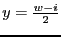whenis odd, andwhenis even.

Figure 9 shows the Xi matrices for the Liberation Code when k=7 and w=7. It may be proven that for all prime w > 2, the Liberation Code for k ≤ w is an MDS code. The complete proof is beyond the scope of this paper, and is instead in an accompanying technical report [Plank07A]. We provide a sketch of the proof at the end of this paper in Section 7.For any given values of k and w, the Xi matrices have a total of kw+k-1 ones. Add this to the kw ones for device P 's matrices, and that makes 2kw+k-1 ones in the CDM. If a coding bit's row of the CDM has o ones, it takes (o-1) XORs to encode that bit from the data bits. Therefore, each coding bit requires an average ofXORs. Optimal is k-1.

The average ones per column of the CDM is, which is roughly two. Optimal is two. Thus, the Liberation codes achieve near optimal performance for both encoding and modification. We explore the notion of optimality in terms of the number of ones in an MDS RAID-6 CDM in Section 6 below. There we will show that the Liberation Codes achieve the lower bound on number of ones in a matrix.

## 3.4 Bit Matrix Scheduling for Decoding

To motivate the need for bit matrix scheduling, consider an example when k=5 and w=5. We encode using the Liberation code, and devices D0 and D1 fail. To decode, we create BDM' by deleting the top 10 rows of the BDM and inverting it. The first 10 rows of this inverted matrix allow us to recalculate D0 and D1 from the surviving devices. This is depicted in Figure 10.Calculating the ten dot products in the straightforward way takes 124 XORs, since there are 134 ones in the matrix. Optimal decoding would take 40 XORs. Now, consider rows 0 and 5 of the matrix which are used to calculate d0,0 and d1,0 respectively. Row 0 has 16 ones, and row 5 has 14 ones, which means that d0,0 and d1,0 may be calculated with 28 XORs in the straightforward manner. However, there are 13 columns in which both rows have ones. Therefore, suppose we first calculate d1,0, which takes 13 XORs, and then calculate d0,0 using the equation:This only takes four additional XOR operations, lowering the total for the two bits from 28 XORs to 17.

This observation leads us to a simple algorithm which we call bit matrix scheduling, for performing a collection of dot products in a bit matrix-vector product more efficiently than simply performing each dot product independently. To describe the algorithm, we use the following assumptions and notation:

• We are multiplying matrix M by vector V to calculate the product vector U. All elements are bits and arithmetic is in GF(2).
• Matrix M has r rows and c columns. The element in row i, column j is denoted M[i,j]. Vector V has c elements denoted  V, ..., V[c-1], and vector p has r elements denoted  U, ..., U[r-1].
• We denote row i of M as Mi.
• From[] is a vector of r integers, each initialized to-1.
• Ones[] is a vector of r integers, initialized so that Ones[i] equals the number of ones in row i of the matrix.
• Sum(i,j) is a c-element bit vector that equals the sum (in GF(2) ) of Mi and Mj.
• Notdone is a set of integers initialized to contain all values in [0..r-1].

The algorithm proceeds in r steps. Each step performs the following operations:

1. Select i such that  i ∈ Notdone and Ones[i] is minimized. Break ties arbitrarily.
2. If From[i] equals-1, then U[i] is calculated to be the XOR of all V[j] such that  M[i,j] = 1. If From[j] does not equal-1, then U[i] is calculated as the XOR of  U[From[i]] and all V[j] such that  M[i,j] + M[From[i],j] = 1.
3. Remove i from Notdone.
4. For all  j ∈ Notdone, calculate x to be one plus the number of ones in Sum(i,j). If  x < Ones[j], then set Ones[j] to x , and From[j] to i.

Thus, if it is more efficient to calculate the product element from another product element than from the original vector, this algorithm makes that happen. When the algorithm operates on the example in Figure 10, it ends up with the following schedule:

• Calculate d1,3 : 7 XORs.
• Calculate d0,3 from d1,3 : 4 XORs.
• Calculate d1,4 from d0,3 : 5 XORs.
• Calculate d0,4 from d1,4 : 4 XORs.
• Calculate d1,0 from d0,4 : 5 XORs.
• Calculate d0,0 from d1,1 : 4 XORs.
• Calculate d1,1 from d0,0 : 4 XORs.
• Calculate d0,1 from d1,1 : 4 XORs.
• Calculate d1,2 from d0,1 : 5 XORs.
• Calculate d0,2 from d1,2 : 4 XORs.

This is a total of 46 XORs, as opposed to 124 without scheduling. An optimal algorithm would decode with 40 XORs.

We note that this algorithm does not always yield an optimal schedule of operations. For example, one can encode using the matrix in Figure 8(a) (EVENODD coding with k=6, w=6 ) with exactly 41 XOR operations by first calculatingand using S in each dot product. When the bit scheduling algorithm is applied to that matrix, however, it is unable to discover this optimization, and in fact yields no improvements in encoding: the dot products take 55 XORs.

However, for decoding with the Liberation Codes, this algorithm improves performance greatly. As an interesting aside, the algorithm derives the optimal schedule for both encoding and decoding using the bit matrix versions of RDP codes, and it improves the performance of both encoding and decoding with Cauchy Reed-Solomon coding. It is an open question to come up with an efficient algorithm that produces optimal schedules for all bit matrix-vector products.

## 3.5 Caching Schedules

The algorithm for bit matrix scheduling, like the inversion of the BDM' matrix, is O(w3). Since w is likely to be relatively small in a RAID-6 system, and since encoding and decoding both involve XORs of O(w2) distinct elements, the inversion and bit scheduling should not add much time to performance of either operation. However, since the total possible number of schedules is bounded by, it is completely plausible to precalculate each of theschedules and cache them for faster encoding and decoding.

# 4 Performance

We have implemented encoding, modification and decoding using all the techniques described in this paper. In all the graphs below, the numbers were generated by instrumenting the implementation and counting the XOR operations. When there is a closed-form expression for a metric (e.g., encoding with RDP, EVENODD, or Liberation codes), we corroborated our numbers with the expression to make sure that they matched.

## 4.1 Performance of Encoding

We measure the performance of encoding as the average number of XOR operations required per coding word. This includes encoding both the P and Q devices. Since optimal encoding is k-1 XORs per coding word, we can normalize by dividing the number of XORs per coding word by k-1 to achieve the overhead of the code: the factor of encoding performance worse than optimal performance. Thus, low values are desirable, the optimal value being one. These values are presented in Figure 11.RDP encoding achieves optimality when k+1 and k+2 are prime numbers. Otherwise, the code is shortened by assuming that there are data devices that hold nothing but zeros [Corbett04]. As the code is asymmetric, the best performance is achieved by assuming that the first w-k devices are zero devices. This is as opposed to EVENODD coding, which performs best when the last w-k devices are zero devices. With both RDP and EVENODD coding, w is a function of k , as smaller w perform better than larger w.

With Liberation codes, this is not the case- larger w perform better than smaller w. For that reason, we plot four values of w in Figure 11. The lines are flat, because the number of XORs per coding word (from Section 3.3) is equal to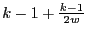, and therefore their factor over optimal is. As such, the codes are asymptotically optimal as w → ∞. As a practical matter though, smaller w require the coding engine to store fewer blocks of data in memory, and may perform better than larger w due to memory and caching effects. The selection of a good w in Liberation Coding thus involves a tradeoff between the fewer XORs required by large w and the reduced memory consumption of small w.

The performance of EVENODD encoding is roughly, which is worse than both RDP and Liberation encoding except when k = w in Liberation Coding and the two perform equally.

The Cauchy Reed-Solomon codes for various w are also included in the graph. Like Liberation Codes, Cauchy Reed-Solomon codes perform better with larger w than with smaller w . However, unlike the other codes, their performance relative to optimal worsens as k grows. It is interesting to note that they outperform EVENODD coding for small k. Since their performance is so much worse than the others, we omit them in subsequent graphs.One of the attractive features of these XOR codes is that if w is chosen to be large enough, then the same code can support any k ≤ w devices. Adding or subtracting devices only involves modification to coding devices P and Q, and does not require re-encoding the entire system as, for example, the X-Code would [Xu99]. For that reason, Figure 12 shows the performance of RDP, EVENODD and Liberation encoding when w is fixed. Since RDP and EVENODD coding require w+1 to be prime, and Liberation coding requires w to be prime, we cannot compare the same values of w, but values that are similar and that can support nearly the same number of data devices. Although RDP outperforms the Liberation codes for larger k, for smaller k, the Liberation codes perform better. Moreover, their performance relative to optimal is fixed for all k, which may ease the act of scheduling coding operations in a distributed storage system.

## 4.2 Performance of Modification

Figure 13 shows the average number of coding bits that must be modified when a data bit is updated. With both EVENODD and RDP coding, this number increases with w, reaching a limit of three as w grows. With Liberation codes, the opposite is true, as the number of modified coding bits is roughly two. Clearly, the Liberation codes outperform the other two in modification performance.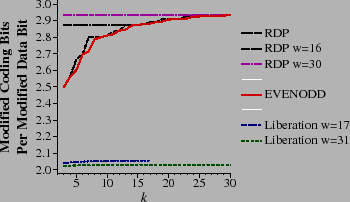## 4.3 Performance of Decoding

For single failures, all RAID-6 systems decode identically. If the failure is in a data device, then it may be decoded optimally from the P device. Otherwise, decoding is identical to encoding. Thus, we only concern ourselves with two-device failures. To test decoding, we measured the performance of decoding for each of thepossible combinations of failures. As with encoding, we measure number of XORs per failed word and present the average value. In Figure 14 we plot the measurements, again as a factor over optimal, which is k-1 XORs per failed word.In general, RDP coding exhibits the best decoding performance, followed by EVENODD coding and then Liberation coding, which decodes at a rate between ten and fifteen percent over optimal. The effectiveness of bit matrix scheduling is displayed in Figure 15, which shows the performance of Liberation decoding without scheduling for w=17 and w=31.Figure 15 clearly shows that without bit scheduling, Liberation codes would be unusable as a RAID-6 technique. It remains a topic of future work to see if the scheduling algorithm of Section 3.4 may be improved further.

## 4.4 Comparison to Reed-Solomon Coding

We do not include a detailed comparison of Liberation Coding to standard Reed-Solomon coding. Instead, in Figure 16 we present measurements of the basic operations of Reed-Solomon coding on three different machines. The first machine is a MacBook Pro with a 2.16 GHz Intel Core 2 Duo processor. The second is a Dell Precision with a 1.5 GHz Intel Pentium processor. The third is a Toshiba Tecra with a 1.73 GHz Intel Pentium processor. On each, we measure the bandwidth of three operations: XOR, multiplication by an arbitrary constant in GF(28) and multiplication by two using Anvin's optimization [Anvin07]. All operations are implemented using the jerasure library presented in Section 5. In particular, multiplication by an arbitrary constant is implemented using a 256 by 256 multiplication table.We may project the performance of standard and optimized Reed-Solomon coding as follows. Let  B be the bandwidth of XOR (in GB/s),  B be the bandwidth of arbitrary multiplication, and  B⊗2 be the bandwidth of multiplication by two. The time to encode one gigabyte of data on k devices using standard Reed-Solomon coding is: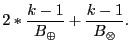This is because the P device is still encoded with parity, and the Q device requires (k-1) multiplications by a constant. The time to encode one megabyte with Anvin's optimization simply substitutes  B with  B⊗2 . Finally, optimal encoding time is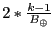, reflecting k-1 XORs per coding word.In Figure 17, we plot the performance of encoding using the bandwidth numbers from Figure 16. For standard Reed-Solomon coding, the performance of decoding is roughly equal to encoding performance. Anvin's optimization improves the performance of encoding roughly by a factor of three. However, it is much worse than the XOR-based codes. Moreover, the optimization does not apply to decoding, which will perform at the same rate as standard Reed-Solomon coding. Thus, we conclude that even with the optimization, Reed-Solomon coding is an unattractive alternative for RAID-6 applications.

Table: Relevent procedures from the Jerasure Coding Library [Plank07B].
 int ** jerasure_bitmatrix_to_schedule(int k, int m, int w, int *bitmatrix); int *** jerasure_generate_schedule_cache(int k, int m, int w, int *bitmatrix); void jerasure_bitmatrix_encode(int k, int m, int w, int *bitmatrix, char **data, char **coding, int totalsize, int B); void jerasure_schedule_encode(int k, int m, int w, int **schedule, char **data, char **coding, int totalsize, int B); int jerasure_bitmatrix_decode(int k, int m, int w, int *bitmatrix, int *erasures, char **data, char **coding, int totalsize, int B); int jerasure_schedule_decode_lazy(int k, int m, int w, int *bitmatrix, int *erasures, char **data, char **coding, int totalsize, int B); int jerasure_schedule_decode_cache(int k, int m, int w, int ***cache, int *erasures, char **data, char **coding, int totalsize, int B); int jerasure_invert_bitmatrix(int *mat, int *inv, int rows); void jerasure_bitmatrix_dotprod(int k, int w, int *bitmatrix_row, int *src_ids, int dest_id, char **data, char **coding, int totalsize, int B); void jerasure_do_scheduled_operations(char **ptrs, int **schedule, int B); int * liberation_coding_bitmatrix(int k, int w); 

# 5 Liberation Coding Library

We have implemented a library in C/C++ to facilitate all Liberation coding operations. It is part of the jerasure library [Plank07B], which implements all manners of matrix and bit matrix coding, including regular Reed-Solomon coding, Cauchy Reed-Solomon coding and Liberation coding. The library is roughly 6000 lines of code and is freely available under the GNU LPL.

Tablelists some of the relevant procedures from the library. In all the procedures, k, w and B are as defined in this paper, m is the number of coding devices (m=2 for RAID-6), data and coding are pointers to data and coding regions, and totalsize is the total number of bytes in each device. Note that totalsize must be a multiple of B and the machine's word size. Bit matrices are represented as linear arrays of integers whose values are either zero or one. The element in row i and column j is in array element ikw + j.

The first procedure creates a schedule from a bit matrix, which may be an encoding or decoding bit matrix. The schedule is an array of operations, where each operation is itself an array of five integers: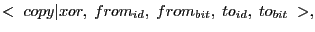where copy|xor specifies whether the operation is to copy data or XOR it, fromid is the id of the source device, frombit is the source bit number (i.e. a number from 0 to w-1 ), toid is the id of the destination device and tobit is the destination bit number. jerasure_generate_schedule_cache() creates a cache of all possible decoding schedules.

The two encoding routines encode using either a bit matrix or a schedule, and the three decoding routines decode using a bit matrix with no schedule, a bit matrix generating a schedule on the fly, or a schedule cache respectively.

jerasure_invert_bitmatrix() inverts a  rows by rows bit matrix, and the two following routines are helper routines for performing bit matrix dot products and scheduled operations respectively. Finally, liberation_coding_bitmatrix generates the Liberation Coding bit matrix defined in Section 3.3 for the given values of k and w.

# 6 Minimal Number of Ones

We state the following properties of RAID-6 codes and  w by w bit matrices [Blaum99,Plank07A]:

• Property #1: Given a RAID-6 code that uses only XORs, this code may be represented by a CDM, which in turn may be specified by the matrices  X0, ..., Xk-1. If the code is MDS, then each Xi must be an invertible  w by w matrix.
• Property #2: Given a MDS RAID-6 code as above, for every i,j such that i ≠ j, the matrix  (Xi + Xj) must be invertible.
• Property #3: If a  w by w matrix is invertible, then it must have at least w ones.
• Property #4: A permutation matrix, Iwπ is a  w by w matrix that has w ones such that there is exactly one one in every row and column of the matrix. Permutation matrices are the only matrices with exactly w ones that are invertible.
• Property #5: Let Iwπ and  Iwπ' be two permutation matrices. Their sum  (Iwπ + Iwπ') is not invertible.

Now consider a RAID-6 code represented by  X0, ..., Xk-1 such that for some i ≠ j, Xi and Xj have exactly w ones each. This code cannot be MDS, because Xi and Xj must be permutation matrices, or they are not invertible. Since they are permutation matrices, their sum is not invertible. Therefore, a RAID-6 code may only have one Xi that has exactly w ones. The other Xj must have more than w ones.

Since the Liberation Codes have one matrix with exactly w ones, and k-1 matrices with w+1 ones, they are minimal RAID-6 matrices. It is an interesting by product of this argument that no MDS RAID-6 code can have optimal modification overhead. The Liberation Codes thereby achieve the lower bound on modification overhead. As an aside, the X-Code [Xu99] does have optimal modification overhead; however the X-Code does not fit the RAID-6 paradigm.

# 7 Sketch of the MDS Proof

First we specify some notation: In the descriptions that follow, <x>w is equal to (x mod w ). If x is negative, <x>w is equal to <w+x>w.

It is a trivial matter to prove that the Xi matrices for Liberation codes are invertible. Moreover, it is easy to prove that  (X0 + Xi) is invertible for 0 < i < w. The challenge in proving the Liberation codes to be MDS lies in the invertibility of  (Xi + Xj) for  0 < i < j < w.

To demonstrate the invertibility of these matrices, we define a class of  w by w matrices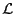to contain all matrices M such that: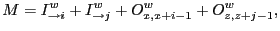with i, j, x and z being subject to the following constraints:

• w is an odd number greater than one.
• 0 ≤ i < j < w.
• GCD(j-i, w) = 1.
• If (j-i) is even:.
• If (j-i) is odd:.

In Figure 18 we show two examples matrices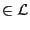. In the first, (j-i) = 4, and <z - x>w = 5, which is indeed. In the second, (j-i) = 1, and <z- x>w = 3, which is.In the technical report, we prove by induction that all matricesare invertible [Plank07A]. Here we demonstrate that in the Liberation codes, when  0 < i < j < w, the matrix. For example, when w=7, (X1 + X5) is equal to the first matrix in Figure 18, and  (X2 + X3) is equal to the second matrix.

From the Liberation code definition in Section 3.3:where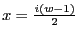and. Therefore, it is in the proper format to be an element of, so long as the constraints are satisfied.

Since w is a prime number greater than two, it must be an odd number greater than one, and GCD(j-i, w) = 1. Now, consider the difference (z- x):When (j-i) is even:When (j-i) is odd:Thus,fits the constraints to be an element of, and is invertible.

# 8 Conclusions/Future Work

In this paper, we have defined a new class of erasure codes, called Liberation Codes, for RAID-6 applications with k data devices. They are parity array codes represented by  w by w bit matrices where w is a prime number ≥ k. Their encoding performance is excellent, achieving a factor ofover optimal. This is an improvement in all cases over EVENODD encoding, and in some cases over RDP encoding. Their decoding performance does not outperform the other two codes, but has been measured to be within 15% of optimal. Their modification overhead is roughly two coding words per modified data word, which is not only an improvement over both EVENODD and RDP coding, but is fact optimal for a RAID-6 code.

In order to make decoding work quickly, we have presented an algorithm for scheduling the XOR operations of a bit matrix-vector product. The algorithm is simple and not effective for all bit matrices, but is very effective for Liberation decoding, reducing the overhead of decoding by a factor of six when w=17, and over eleven when w=31.

Besides comparing Liberation Codes to RDP and EVENODD coding, we assess their performance in comparison to Reed-Solomon coding. In all cases, they outperform Reed-Solomon coding greatly. We have written a freely-available library to facilitate the use of Liberation Codes in RAID-6 applications.

In sum, Liberation Codes are extremely attractive alternatives to other RAID-6 implementations. We anticipate that their simple structure, excellent performance and availability in library form will make them popular with RAID-6 implementors.

Our future work in this project is proceeding along three lines. First, the Liberation Codes are only defined for prime w. We are currently working to discover optimal RAID-6 codes for non-prime w. In particular, values of w which are powers of two are quite attractive. Our search has been based on Monte-Carlo techniques, attempting to build good matrices from smaller matrices and to improve on the best current matrices by modifying them slightly. Currently, the search has yielded optimal matrices for nearly every value of k ≤ 8 and w ≤ 32. We will continue to explore these constructions.

Second, we are looking to construct better bit matrix scheduling algorithms. Although the Liberation decoding cannot be improved much further, it is clear from our current algorithm's inability to schedule EVENODD coding effectively that further refinements are available. In its simplest case, bit scheduling is equivalent to common subexpression removal in compiler systems [Aho86,Briggs97,Cocke70]. Huang et al have recently reduced this case to an NP-complete problem and give a heuristic based on matching to solve it [Huang07B]. However, the fact that one plus one equals zero in GF(2) means that there are additional ways to improve performance, one of which is illustrated by the scheduling algorithm in Section 3.4. We are exploring these and other methodologies to further probe into the problem.

Finally, we have yet to explore how Liberation Codes may extrapolate systems that need to tolerate more failures. We plan to probe into minimal conditions for general MDS codes based on bit matrices such as those presented in Section 6, to see if the Liberation Code construction has application for larger classes of failures.

# 9 Availability

The jerasure library is available at https://www.cs.utk.edu/~plank/plank/papers/CS-07-603.html.

# 10 Acknowledgements

This material is based upon work supported by the National Science Foundation under grant CNS-0615221. The author would like to thank Adam Buchsbaum for working to prove the Liberation Codes' MDS property, Lihao Xu for helpful discussions, and the program chairs and reviewers for their constructive comments.

## References

[Wylie07] J. J. Wylie and R. Swaminathan, "Determining Fault Tolerance of XOR-based Erasure Codes Efficiently," DSN-2007: The International Conference on Dependable Systems and Networks, IEEE, Edinburgh, Scotland, June, 2007.

The RAID-6 Liberation Codes

This document was generated using the LaTeX2HTML translator Version 2002-2-1 (1.71)

Copyright © 1993, 1994, 1995, 1996, Nikos Drakos, Computer Based Learning Unit, University of Leeds.
Copyright © 1997, 1998, 1999, Ross Moore, Mathematics Department, Macquarie University, Sydney.

The command line arguments were:
latex2html -split 0 -show_section_numbers -local_icons -no_navigation plank_html

The translation was initiated by James Plank on 2008-01-09. References were done by hand, and getting rid of a lot of the math was done with the following shell script:

 `sed 's/.nbsp;

James Plank 2008-01-09